Question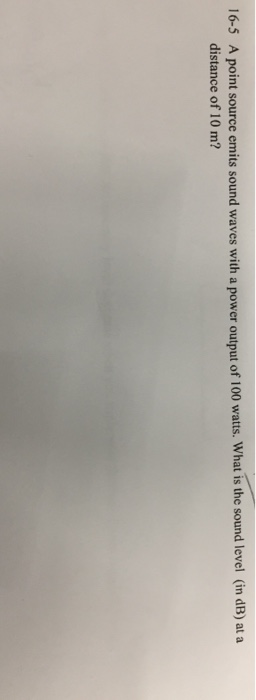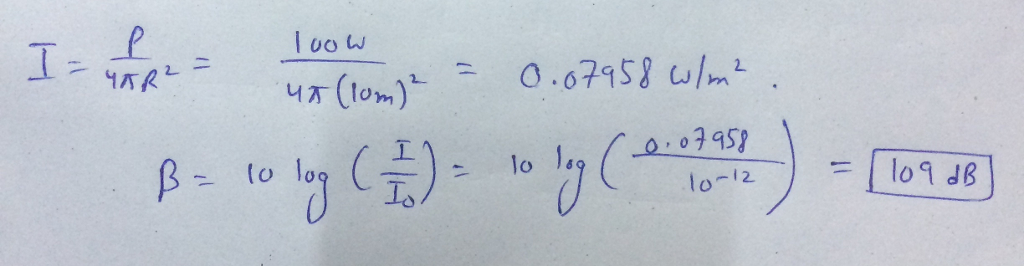#### Earn Coins

Coins can be redeemed for fabulous gifts.

Similar Homework Help Questions
• ### 2 parts thx :) 1. A point source emits sound waves isotropically. A sound meter measures...

2 parts thx :) 1. A point source emits sound waves isotropically. A sound meter measures a sound level of 51 dB at location C and a sound level of 48.47 at location D, a distance of 16 m from location C. The two locations C and D and the point source are all located along the same line. How far from location C is the point source? Give your answer in m, though enter only the numerical part in...

• ### An outside loudspeaker (considered a small source) emits sound waves with a power output of 111...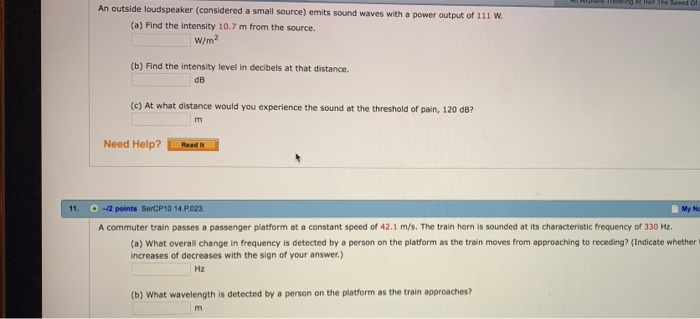An outside loudspeaker (considered a small source) emits sound waves with a power output of 111 w (a) Find the intensity 10.7 m from the source W/m2 (b) Find the intensity level in decibels at that distance. dB (c) At what distance would you experience the sound at the threshold of pain, 120 dB? Need Help? Read 11. -/2 points SerCP10 14 P023 My No A commuter train passes a passenger platform at a constant speed of 42.1 m/s. The...

• ### An outside loudspeaker (considered a small source) emits sound waves with a power output of 111...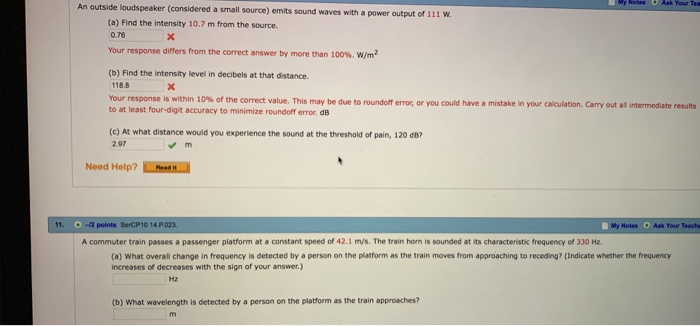An outside loudspeaker (considered a small source) emits sound waves with a power output of 111 W (a) Find the intensity 10.7 m from the source. 0.76 Your response differs from the correct answer by more than 100%, w/m? (b) Find the intensity level in decibels at that distance. 118.8 Your response is within 10% of the correct value. This may be due to roundoff error, or you could have a mistake in your calculation Cary out all inter to...

• ### 5. A point source emits a sound with an average power output of 80.0w. a) What...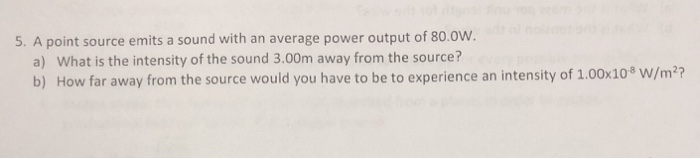5. A point source emits a sound with an average power output of 80.0w. a) What is the intensity of the sound 3.00m away from the source? b) How far away from the source would you have to be to experience an intensity of 1.00x108 W/m2?

• ### QUESTION The power output of a sound system is increased by a factor of 25. By...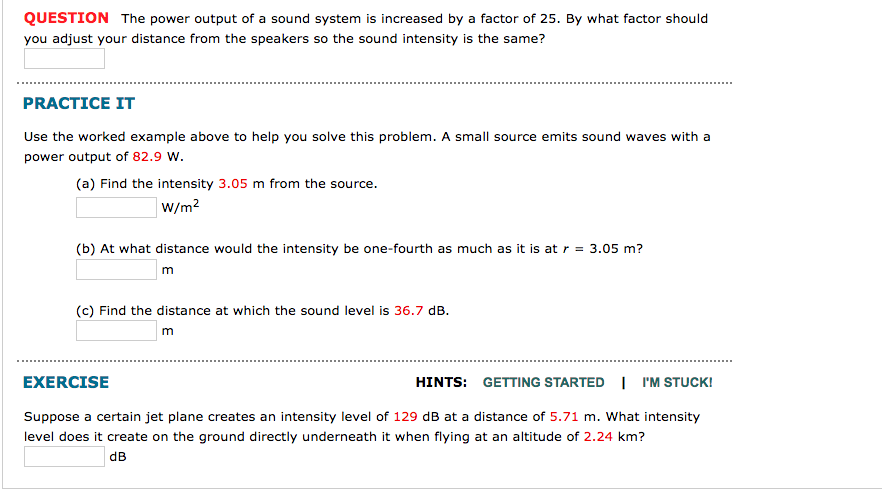QUESTION The power output of a sound system is increased by a factor of 25. By what factor should you adjust your distance from the speakers so the sound intensity is the same? PRACTICE IT Use the worked example above to help you solve this problem. A small source emits sound waves with a power output of 82.9 w. (a) Find the intensity 3.05 m from the source. W/m2 (b) At what distance would the intensity be one-fourth as much...

• ### An electronic point source emits sound isotropically at a frequency of 2 × 103 Hz and...

An electronic point source emits sound isotropically at a frequency of 2 × 103 Hz and a power of 27 watts. A small microphone has an area of 0.77 cm2 and is located 175 meters from the point source. a: How many minutes will it take for the microphone to recieve 0.2 Joules from this sound? b: What is the sound intensity level at the microphone from this point source? c: What would be the sound intensity level at the...

• ### Sound waves at ultrasonic frequencies attenuate much faster than audible sound waves. The sound intensity level...

Sound waves at ultrasonic frequencies attenuate much faster than audible sound waves. The sound intensity level of a point source of ultrasonic sound waves is measured as 100 dB at a distance of 1.00 m from the source and 65 dB at 2.00 m. Assuming the waves spread in all directions, what is the attenuation coefficient alpha? [Answer in 1/m to within 5%]

• ### Sound waves at ultrasonic frequencies attenuate much faster than audible sound waves. The sound intensity level...

Sound waves at ultrasonic frequencies attenuate much faster than audible sound waves. The sound intensity level of a point source of ultrasonic sound waves is measured as 100 dB at a distance of 1.00 m from the source and 65 dB at 2.00 m. Assuming the waves spread in all directions, what is the attenuation coefficient alpha? [Answer in 1/m to within 5%]

• ### title: ergonomics A point source in a free field (no reflected sound waves) produces a sound...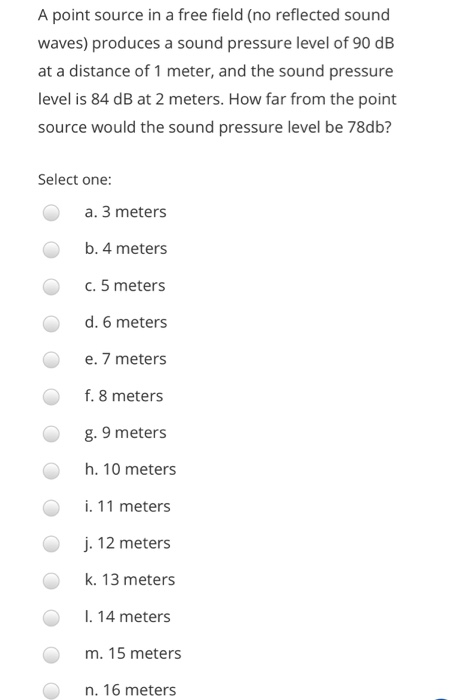title: ergonomics A point source in a free field (no reflected sound waves) produces a sound pressure level of 90 dB at a distance of 1 meter, and the sound pressure level is 84 dB at 2 meters. How far from the point source would the sound pressure level be 78db? Select one: O a. 3 meters b. 4 meters c. 5 meters d. 6 meters e. 7 meters f. 8 meters g. 9 meters h. 10 meters OOO OOO...

• ### QUESTION The power output of a sound system is increased by a factor of 25. By...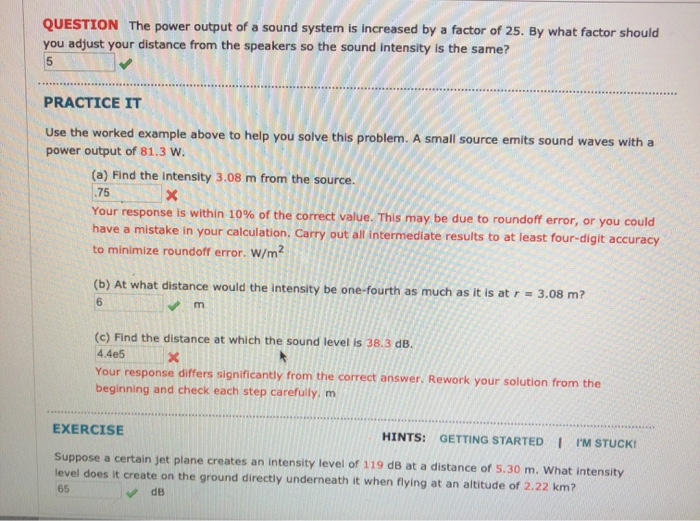QUESTION The power output of a sound system is increased by a factor of 25. By what factor should you adjust your distance from the speakers so the sound intensity is the same? . PRACTICE IT .............. Use the worked example above to help you solve this problem. A small source emits sound waves with a power output of 81.3 W. (a) Find the intensity 3.08 m from the source. . 75X Your response is within 10% of the correct...